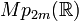# Metaplectic group

Let$m$ be a natural number. The metaplectic group$Mp(2m,\R)$, also denoted$Mp_{2m}(\R)$, is defined as the unique double cover of the symplectic group$Sp(2m,\R)$ over the field of real numbers. This makes sense because the fundamental group of any$Sp(2m,\R)$ is an infinite cyclic group and hence has a unique subgroup of index two.
Let$m$ be a natural number and$F$ be a local field other than the field of complex numbers. The group$Mp(2m,F)$ is the unique perfect central extension with the symplectic group$Sp(2m,F)$ as the quotient group and cyclic group:Z2 as the base normal subgroup (in this case, central subgroup).